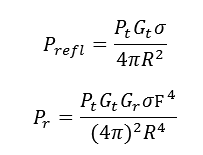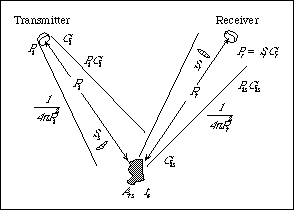# Radar Equation Calculator

This CalcTown calculator calculates the power  returning to the receiver antenna.

m2
m2

#### Result

W
WClick here to view image

Where,

Pt = Transmitted Power

Pr = Power returning to the Receiver Antenna (1-way radar equation)

Prefl = Reflected signal from target

Gt = Gain of the Transmitting Antenna

Gr = Effective Aperture of the Receiving Antenna

σ = Radar Cross-section or Scattering co-efficient of target

F = Pattern Propagation Factor

R = Range (distance from transmitter or receiver to target)